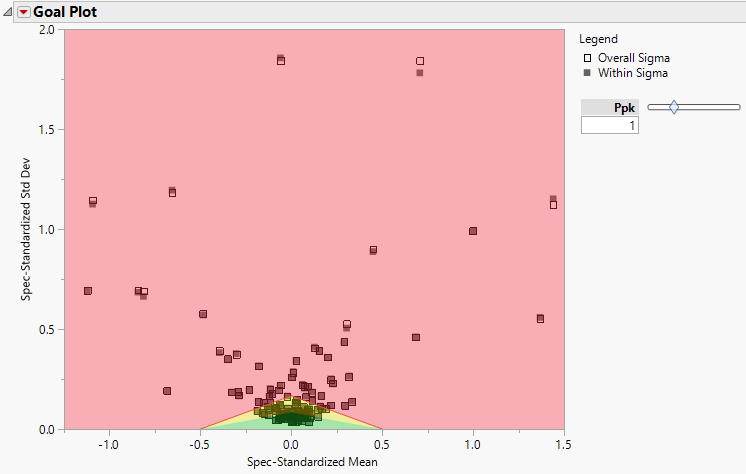Publication date: 11/10/2021

## Goal Plot

The Goal Plot shows, for each variable, the spec-normalized mean shift on the x-axis, and the spec-normalized standard deviation on the y-axis. It is useful for getting a quick, summary view of how the variables are conforming to specification limits. By default, the Goal Plot shows only those points for each column that are calculated using the overall sigma. Hover over each point to view the variable name and the sigma method used to calculate the point. See Goal Plot for more information about the calculation of the coordinates for the Goal Plot.

Note: Process variables with distributions other than Normal are not plotted on the Goal Plot.

### Goal Plot Points

Points on the Goal Plot correspond to columns, not rows. Selecting a point in the Goal Plot selects the corresponding column in the data table. If process importance values are specified, the goal plot points are sized by importance.

Hover over a point in the Goal Plot to view a control chart for that process. Click the control chart to launch Control Chart Builder with the corresponding control chart and capability report.

Note: A control chart is not available for a process if the unbiased pooled standard deviation is chosen as the within-group variation statistic for that process.

The points on the Goal Plot are also linked to the rows of the Goal Plot Summary Table, where each row corresponds to a column. You can select a point in the Goal Plot, right-click, and apply row states. These row states are applied to the rows of the Goal Plot Summary Table. Row states that you apply in the Goal Plot Summary Table are reflected in the Goal Plot. To see this table, select Make Goal Plot Summary Table from the Process Capability red triangle menu. See Make Goal Plot Summary Table.

Tip: If you hide a point in the Goal Plot, you can show the point again by changing the corresponding row state in the Goal Plot Summary Table.

### Goal Plot Triangle

The goal plot triangle appears in the center of the bottom of the Goal Plot. The slider to the right of the plot enables you to adjust the size of goal triangle in the plot.

By default, the Ppk slider and the value beneath it are set to Ppk = 1. This approximates a non-conformance rate of 0.0027, if the distribution is normal. The goal triangle represents the Ppk shown in the box. To change the Ppk value, move the slider or enter a number in the box.

JMP gives the Goal Plot in terms of Ppk values by default. You can change this preference at File > Preferences > Platforms > Process Capability. When the AIAG (Ppk) Labeling preference is unchecked, all of the Ppk labeling is changed to Cpk labeling, including the label of the slider to the right of the goal plot.

### Goal Plot Options

The Goal Plot red triangle menu has the following options:

Show Within Sigma Points

Shows or hides the points calculated using the within sigma estimate.

Show Within or Between-and-Within Sigma Points

(Available only when Calculate Between-and-Within Capability is selected for at least one process in the launch window.) Shows or hides the points calculated using the within sigma estimate or, if specified, the between-and-within sigma estimate.

Show Overall Sigma Points

Shows or hides the points calculated using the overall sigma estimate.

Shows or hides the Ppk level shading (Figure 7.11). When you select Shade Levels, shaded areas appear in the plot. The shaded areas depend on the relationship between p and Ppk, with p representing the value shown in the box beneath Ppk:

Points in the red area have Ppk < p.

Points in the yellow area have p < Ppk < 2p.

Points in the green area have 2p < Ppk.

Label Within Sigma Points

Shows or hides labels for points calculated using the within sigma estimate.

Label Within or Between-and-Within Sigma Points

(Available only when Calculate Between-and-Within Capability is selected for at least one process in the launch window.) Shows or hides labels for points calculated using the within sigma estimate or, if specified, the between-and-within sigma estimate.

Label Overall Sigma Points

Shows or hides labels for points calculated using the overall sigma estimate.

Defect Rate Contour

Shows or hides a contour representing a specified defect rate.

Figure 7.11 shows the Goal Plot for the entire data set for the Semiconductor Capability.jmp sample data table after selecting Shade Levels and Show Within Sigma Points from the Goal Plot red triangle menu.

Figure 7.11 Goal Plot### One-Sided or Missing Specification Limits

When there is only one specification limit for a column, markers and colors are used in the following ways:

If only the upper specification limit (USL) is specified, the point on the Goal Plot is represented by a right-pointing triangle and is colored blue.

If only the lower specification limit (LSL) is specified, the point on the Goal Plot is represented by a left-pointing triangle and is colored red.

If at least one process has only an upper specification limit, the right half of the goal triangle is blue.

If at least one process has only a lower specification limit, the left half of the goal triangle is red.

Processes with only an upper specification limit are represented by blue and should be compared to the blue (right) side of the goal triangle. Processes with only a lower specification limit are represented by red and should be compared to the red (left) side of the goal triangle. For more information about how the coordinates of points are calculated, see Goal Plot.